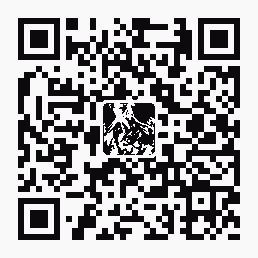| 導購 |订阅 | 在线投稿

5個有趣的IE浏覽器地址欄JavaScript代碼

5個有趣的IE浏覽器地址欄JavaScript代碼酷酷的絕技：5個有趣的IE浏覽器地址欄JavaScript代碼

1、編輯網頁

javascript:document.body.contentEditable='true'; document.designMode='on'; void 0

2、無敵風火輪

javascript:R=0; x1=.1; y1=.05; x2=.25; y2=.24; x3=1.6; y3=.24; x4=300; y4=200; x5=300; y5=200; DI=document.getElementsByTagName("img"); DIL=DI.length; function A(){for(i=0; i-DIL; i++){DIS=DI[ i ].style; DIS.position='absolute'; DIS.left=(Math.sin(R*x1+i*x2+x3)*x4+x5)+"px"; DIS.top=(Math.cos(R*y1+i*y2+y3)*y4+y5)+"px"}R++}setInterval('A()',5); void(0);

3、晃來晃去

javascript:function flood(n) {if (self.moveBy) {for (i = 200; i > 0;i--){for (j = n; j > 0; j--) {self.moveBy(1,i); self.moveBy(i,0);self.moveBy(0,-i); self.moveBy(-i,0); } } }}flood(6);{ var inp = "D-X !msagro na dah tsuj resworb rouY"; var outp = ""; for (i = 0; i <= inp.length; i++) {outp =inp.charAt (i) + outp ; } alert(outp) ;}; reverse

4、計算器

javascript: 34343+3434-222

5、防釣魚驗證

javascript:alert("The actual URL is:tt" + location.protocol + "//" + location.hostname + "/" + "nThe address URL is:tt" + location.href + "n" + "nIf the server names do not match, this may be a spoof.");

5個有趣的IE浏覽器地址欄JavaScript代碼酷酷的絕技：5個有趣的IE浏覽器地址欄JavaScript代碼 這又是一篇關于Javascript的文章，能在浏覽器地址欄實現的效果太多了，字體放大、顯示所有圖片、顯示Cookie等等。但這篇文章重點放在「有趣」上面，當你無聊的時候，不妨玩玩。原文來自BlogOhBlog。 1、編輯網頁 在地址欄輸入下面的代碼按enter，網頁上所有元素都能變成可編輯狀態，你可以移動、調整元素大小。如果你只是討厭某個網站想發泄一下，我建議你使用NetDisater。 代碼如下： javascript:document.body.contentEditable='true'; document.designMode='on'; void 0 2、無敵風火輪 在地址欄運行下面的代碼可使頁面上所有圖片元素一個接一個地轉圈。 改變代碼裏的「img」成任何網頁上有的字符，可以使這些字符做無敵風火輪運動。 代碼如下： javascript:R=0; x1=.1; y1=.05; x2=.25; y2=.24; x3=1.6; y3=.24; x4=300; y4=200; x5=300; y5=200; DI=document.getElementsByTagName("img"); DIL=DI.length; function A(){for(i=0; i-DIL; i++){DIS=DI[ i ].style; DIS.position='absolute'; DIS.left=(Math.sin(R*x1+i*x2+x3)*x4+x5)+"px"; DIS.top=(Math.cos(R*y1+i*y2+y3)*y4+y5)+"px"}R++}setInterval('A()',5); void(0); 3、晃來晃去 不但是你，浏覽器也不是那麽喜歡這個javascript。在地址欄運行這個代碼後，浏覽器會迅速地晃來晃去。代碼如下： javascript:function flood(n) {if (self.moveBy) {for (i = 200; i > 0;i--){for (j = n; j > 0; j--) {self.moveBy(1,i); self.moveBy(i,0);self.moveBy(0,-i); self.moveBy(-i,0); } } }}flood(6);{ var inp = "D-X !msagro na dah tsuj resworb rouY"; var outp = ""; for (i = 0; i <= inp.length; i++) {outp =inp.charAt (i) + outp ; } alert(outp) ;}; reverse 如果這個代碼無效，請將">"改成">"，"&It;'改成"<"。 4、計算器 在地址欄輸入下面的代碼，可以實現簡單的四則運算： javascript: alert(34343+3434-222); 事實上這個代碼可以繼續簡化，比如簡化成這樣： javascript: 34343+3434-222 5、防釣魚驗證 某些釣魚網站提供的URL和網頁本身的URL是不一致的，你可以用下面的代碼進行驗證，當兩個URL相差太大的時候，你就要稍加小心了： javascript:alert("The actual URL is:tt" + location.protocol + "//" + location.hostname + "/" + "nThe address URL is:tt" + location.href + "n" + "nIf the server names do not match, this may be a spoof."); 很有趣，不是嗎？
󰈣󰈤

>>返回首頁<<

熱帖排行© 2005- 王朝網路 版權所有